# AP Chemistry : Thermodynamics

## Example Questions

### Example Question #8 : Cell Potential Under Non Standard Conditions

Calculate the standard cell potential of the following reaction:

3 F2 (g) + 2 Au (s) -> 6 F- (aq) + 2 Au3+

Given:

F2 (g) + 2 e- -> 2 F- (aq)                                                  Eo = 2.87 V

Au3+(aq)+ 3 e--> Au (s)                                                  Eo = 1.50 V

-1.37 V

4.37 V

1.37 V

5.61 V

-5.61 V

1.37 V

Explanation:

Eocell  = Eo cathode - Eoanode

Eocell = 2.87 – (1.50) = 1.37 V

### Example Question #9 : Cell Potential Under Non Standard Conditions

Determine the Ecell for the following reaction at 25 C:

Zn (s) + 2 VO2+ (aq) + 4 H+ -> 2 VO2+  (aq) +  Zn2+(aq) + 2 H2O (l)

Given that:

VO2+ (aq)  +  2 H+ (aq) + e- ->  VO2+(aq) + H2O (l)        Eo = 1.00 V

Zn2+ (aq)  + 2 e-->  Zn (s)                                            Eo = -0.76 V

And

[ VO2+] = 2.0 M; [H+] = 0.50 M; [VO2+] = 1.0 x 10-2M; [Zn2+] = 1.0 x 10-1M

1.76 V

1.89 V

0.95 V

2.41 V

3.71 V

1.89 V

Explanation: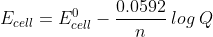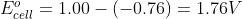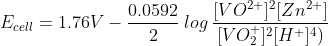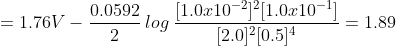### Example Question #1 : Electrolysis And Faraday's Law

How many grams of Cr can be obtained by the electrolysis of a Cr(NO3)3 if 10 amps are passed through the cell for 6 hours?

38.8 g

56.3 g

19.4 g

12.5 g

103 g

38.8 g

Explanation: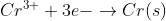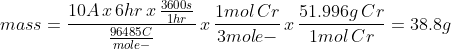### Example Question #2 : Electrolysis And Faraday's Law

How much Al would be precipitated if an AlCl3 solution was electrolyzed for 1.00 hours with 5.00 amps?

3.36 g

1.68 g

1.25 g

0.84 g

6.72 g

1.68 g

Explanation: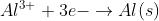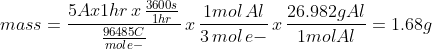### Example Question #3 : Electrolysis And Faraday's Law

What current must be past through a solution of AlCl3 for 1.19 hours to produce 40g of solid Al?

100.  amps

125   amps

75.3  amps

10.5 amps

50.0  amps

100.  amps

Explanation: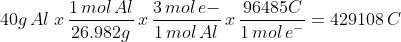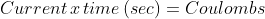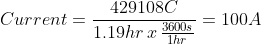### Example Question #4 : Electrolysis And Faraday's Law

In the electrolysis of CaCl2 a 1.15 amp current is passed through the cell for 5.0 hours.  How much Ca is produced?

2.2 g

18.3 g

4.3 g

8.6 g

1.1 g

4.3 g

Explanation: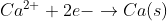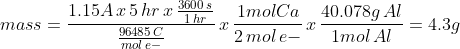### Example Question #5 : Electrolysis And Faraday's Law

How long would it take to electroplate 28.3g of silver at a constant current of 2.0 amps from a solution of silver nitrate?

105 min

422 min

53 min

844 min

211 min

211 min

Explanation: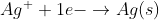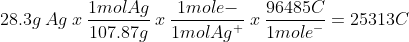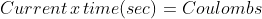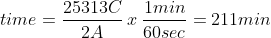### Example Question #3 : Help With Anodes And Cathodes

Consider the following electrolytic cell: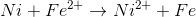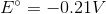What happens at the anode in the electrolytic cell?

Iron is reduced

Iron is oxidized

Nickel is oxidized

Nickel is reduced

Nickel is oxidized

Explanation:

It does not matter if the cell is galvanic or electrolytic; oxidation will always take place at the anode. This means that the nickel loses two electrons and is oxidized at the anode to generate nickel ions.Nickel ions and iron are products, and are neither oxidized nor reduced during the reaction. Iron ions are reduced at the cathode to generate the iron product.

### Example Question #1 : Galvanic (Voltai) And Elecrolytic Cells

Consider the following reaction in a galvanic cell: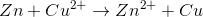Which of the following takes place at the anode?

Zinc is oxidized

Zinc is reduced

No reaction takes place at the anode

Copper is oxidized

Copper is reduced

Zinc is oxidized

Explanation:

Oxidation takes place at the anode and reduction takes place at the cathode. You can remember this with the pneumonic "An Ox, Red Cat."

In the equation, zinc loses electrons. It goes from a neutral, elemental charge to a charge of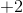. Since electrons are negative, a loss of electrons will cause an increase in charge. Because zinc loses electrons, it is oxidized. This will take place at the anode.

### Example Question #1 : Galvanic (Voltai) And Elecrolytic Cells

Toward which pole do electrons travel in a galvanic cell?

Towards the cathode

Electrons do not travel; only the protons travel

Towards the anode

Towards the negative pole